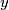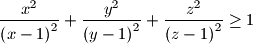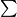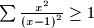### IMO Shortlist 2008 problem A2

Kvaliteta:
Avg: 1,5
Težina:
Avg: 6,0
(i) If$x$,$y$ and$z$ are three real numbers, all different from$1$, such that$xyz = 1$, then prove that.
(With the$\sum$ sign for cyclic summation, this inequality could be rewritten as$\sum \frac {x^{2}}{\left(x - 1\right)^{2}} \geq 1$.)

(ii) Prove that equality is achieved for infinitely many triples of rational numbers$x$,$y$ and$z$.

Author: Walther Janous, Austria
Izvor: Međunarodna matematička olimpijada, shortlist 2008